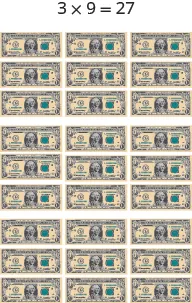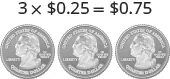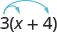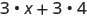Prealgebra 2e

# 7.3Distributive Property

Prealgebra 2e7.3 Distributive Property

### Learning Objectives

By the end of this section, you will be able to:

• Simplify expressions using the distributive property
• Evaluate expressions using the distributive property

### Be Prepared 7.7

Before you get started, take this readiness quiz.

Multiply: $3(0.25).3(0.25).$
If you missed this problem, review Example 5.15

### Be Prepared 7.8

Simplify: $10−(−2)(3).10−(−2)(3).$
If you missed this problem, review Example 3.51

### Be Prepared 7.9

Combine like terms: $9y+17+3y−2.9y+17+3y−2.$
If you missed this problem, review Example 2.22.

### Simplify Expressions Using the Distributive Property

Suppose three friends are going to the movies. They each need $9.25;9.25;$ that is, $99$ dollars and $11$ quarter. How much money do they need all together? You can think about the dollars separately from the quarters.They need $33$ times $9,9,$ so $27,27,$ and $33$ times $11$ quarter, so $7575$ cents. In total, they need $27.75.27.75.$

If you think about doing the math in this way, you are using the Distributive Property.

### Distributive Property

If $a,b,ca,b,c$ are real numbers, then

$a(b+c)=ab+aca(b+c)=ab+ac$

Back to our friends at the movies, we could show the math steps we take to find the total amount of money they need like this:

$3(9.25) 3(9+0.25) 3(9)+3(0.25) 27+0.75 27.75 3(9.25) 3(9+0.25) 3(9)+3(0.25) 27+0.75 27.75$

In algebra, we use the Distributive Property to remove parentheses as we simplify expressions. For example, if we are asked to simplify the expression $3(x+4),3(x+4),$ the order of operations says to work in the parentheses first. But we cannot add $xx$ and $4,4,$ since they are not like terms. So we use the Distributive Property, as shown in Example 7.17.

### Example 7.17

Simplify: $3(x+4).3(x+4).$

### Try It 7.33

Simplify: $4(x+2).4(x+2).$

### Try It 7.34

Simplify: $6(x+7).6(x+7).$

Some students find it helpful to draw in arrows to remind them how to use the Distributive Property. Then the first step in Example 7.17 would look like this:### Example 7.18

Simplify: $6(5y+1).6(5y+1).$

### Try It 7.35

Simplify: $9(3y+8).9(3y+8).$

### Try It 7.36

Simplify: $5(5w+9).5(5w+9).$

The distributive property can be used to simplify expressions that look slightly different from $a(b+c).a(b+c).$ Here are two other forms.

### Distributive Property

If $a,b,ca,b,c$ are real numbers, then

$a(b+c)=ab+aca(b+c)=ab+ac$

Other forms

$a(b−c)=ab−aca(b−c)=ab−ac$
$(b+c)a=ba+ca(b+c)a=ba+ca$

### Example 7.19

Simplify: $2(x−3).2(x−3).$

### Try It 7.37

Simplify: $7(x−6).7(x−6).$

### Try It 7.38

Simplify: $8(x−5).8(x−5).$

Do you remember how to multiply a fraction by a whole number? We’ll need to do that in the next two examples.

### Example 7.20

Simplify: $34(n+12).34(n+12).$

### Try It 7.39

Simplify: $25(p+10).25(p+10).$

### Try It 7.40

Simplify: $37(u+21).37(u+21).$

### Example 7.21

Simplify: $8(38x+14).8(38x+14).$

### Try It 7.41

Simplify: $6(56y+12).6(56y+12).$

### Try It 7.42

Simplify: $12(13n+34).12(13n+34).$

Using the Distributive Property as shown in the next example will be very useful when we solve money applications later.

### Example 7.22

Simplify: $100(0.3+0.25q).100(0.3+0.25q).$

### Try It 7.43

Simplify: $100(0.7+0.15p).100(0.7+0.15p).$

### Try It 7.44

Simplify: $100(0.04+0.35d).100(0.04+0.35d).$

In the next example we’ll multiply by a variable. We’ll need to do this in a later chapter.

### Example 7.23

Simplify: $m(n−4).m(n−4).$

### Try It 7.45

Simplify: $r(s−2).r(s−2).$

### Try It 7.46

Simplify: $y(z−8).y(z−8).$

The next example will use the ‘backwards’ form of the Distributive Property, $(b+c)a=ba+ca.(b+c)a=ba+ca.$

### Example 7.24

Simplify: $(x+8)p.(x+8)p.$

### Try It 7.47

Simplify: $(x+2)p.(x+2)p.$

### Try It 7.48

Simplify: $(y+4)q.(y+4)q.$

When you distribute a negative number, you need to be extra careful to get the signs correct.

### Example 7.25

Simplify: $−2(4y+1).−2(4y+1).$

### Try It 7.49

Simplify: $−3(6m+5).−3(6m+5).$

### Try It 7.50

Simplify: $−6(8n+11).−6(8n+11).$

### Example 7.26

Simplify: $−11(4−3a).−11(4−3a).$

### Try It 7.51

Simplify: $−5(2−3a).−5(2−3a).$

### Try It 7.52

Simplify: $−7(8−15y).−7(8−15y).$

In the next example, we will show how to use the Distributive Property to find the opposite of an expression. Remember, $−a=−1·a.−a=−1·a.$

### Example 7.27

Simplify: $−(y+5).−(y+5).$

### Try It 7.53

Simplify: $−(z−11).−(z−11).$

### Try It 7.54

Simplify: $−(x−4).−(x−4).$

Sometimes we need to use the Distributive Property as part of the order of operations. Start by looking at the parentheses. If the expression inside the parentheses cannot be simplified, the next step would be multiply using the distributive property, which removes the parentheses. The next two examples will illustrate this.

### Example 7.28

Simplify: $8−2(x+3).8−2(x+3).$

### Try It 7.55

Simplify: $9−3(x+2).9−3(x+2).$

### Try It 7.56

Simplify: $7x−5(x+4).7x−5(x+4).$

### Example 7.29

Simplify: $4(x−8)−(x+3).4(x−8)−(x+3).$

### Try It 7.57

Simplify: $6(x−9)−(x+12).6(x−9)−(x+12).$

### Try It 7.58

Simplify: $8(x−1)−(x+5).8(x−1)−(x+5).$

### Evaluate Expressions Using the Distributive Property

Some students need to be convinced that the Distributive Property always works.

In the examples below, we will practice evaluating some of the expressions from previous examples; in part , we will evaluate the form with parentheses, and in part we will evaluate the form we got after distributing. If we evaluate both expressions correctly, this will show that they are indeed equal.

### Example 7.30

When $y=10y=10$ evaluate: $6(5y+1)6(5y+1)$ $6·5y+6·1.6·5y+6·1.$

### Try It 7.59

Evaluate when $w=3:w=3:$ $5(5w+9)5(5w+9)$ $5·5w+5·9.5·5w+5·9.$

### Try It 7.60

Evaluate when $y=2:y=2:$ $9(3y+8)9(3y+8)$ $9·3y+9·8.9·3y+9·8.$

### Example 7.31

When $y=3,y=3,$ evaluate $−2(4y+1)−2(4y+1)$ $−2·4y+(−2)·1.−2·4y+(−2)·1.$

### Try It 7.61

Evaluate when $n=−2:n=−2:$ $−6(8n+11)−6(8n+11)$ $−6·8n+(−6)·11.−6·8n+(−6)·11.$

### Try It 7.62

Evaluate when $m=−1:m=−1:$ $−3(6m+5)−3(6m+5)$ $−3·6m+(−3)·5.−3·6m+(−3)·5.$

### Example 7.32

When $y=35y=35$ evaluate $−(y+5)−(y+5)$ and $−y−5−y−5$ to show that $−(y+5)=−y−5.−(y+5)=−y−5.$

### Try It 7.63

Evaluate when $x=36:x=36:$ $−(x−4)−(x−4)$ $−x+4−x+4$ to show that $−(x−4)=−x+4.−(x−4)=−x+4.$

### Try It 7.64

Evaluate when $z=55:z=55:$ $−(z−10)−(z−10)$ $−z+10−z+10$ to show that $−(z−10)=−z+10.−(z−10)=−z+10.$

### Section 7.3 Exercises

#### Practice Makes Perfect

Simplify Expressions Using the Distributive Property

In the following exercises, simplify using the distributive property.

90.

$4 ( x + 8 ) 4 ( x + 8 )$

91.

$3 ( a + 9 ) 3 ( a + 9 )$

92.

$8 ( 4 y + 9 ) 8 ( 4 y + 9 )$

93.

$9 ( 3 w + 7 ) 9 ( 3 w + 7 )$

94.

$6 ( c − 13 ) 6 ( c − 13 )$

95.

$7 ( y − 13 ) 7 ( y − 13 )$

96.

$7 ( 3 p − 8 ) 7 ( 3 p − 8 )$

97.

$5 ( 7 u − 4 ) 5 ( 7 u − 4 )$

98.

$1 2 ( n + 8 ) 1 2 ( n + 8 )$

99.

$1 3 ( u + 9 ) 1 3 ( u + 9 )$

100.

$1 4 ( 3 q + 12 ) 1 4 ( 3 q + 12 )$

101.

$1 5 ( 4 m + 20 ) 1 5 ( 4 m + 20 )$

102.

$9 ( 5 9 y − 1 3 ) 9 ( 5 9 y − 1 3 )$

103.

$10 ( 3 10 x − 2 5 ) 10 ( 3 10 x − 2 5 )$

104.

$12 ( 1 4 + 2 3 r ) 12 ( 1 4 + 2 3 r )$

105.

$12 ( 1 6 + 3 4 s ) 12 ( 1 6 + 3 4 s )$

106.

$r ( s − 18 ) r ( s − 18 )$

107.

$u ( v − 10 ) u ( v − 10 )$

108.

$( y + 4 ) p ( y + 4 ) p$

109.

$( a + 7 ) x ( a + 7 ) x$

110.

$−2 ( y + 13 ) −2 ( y + 13 )$

111.

$−3 ( a + 11 ) −3 ( a + 11 )$

112.

$−7 ( 4 p + 1 ) −7 ( 4 p + 1 )$

113.

$−9 ( 9 a + 4 ) −9 ( 9 a + 4 )$

114.

$−3 ( x − 6 ) −3 ( x − 6 )$

115.

$−4 ( q − 7 ) −4 ( q − 7 )$

116.

$−9 ( 3 a − 7 ) −9 ( 3 a − 7 )$

117.

$−6 ( 7 x − 8 ) −6 ( 7 x − 8 )$

118.

$− ( r + 7 ) − ( r + 7 )$

119.

$− ( q + 11 ) − ( q + 11 )$

120.

$− ( 3 x − 7 ) − ( 3 x − 7 )$

121.

$− ( 5 p − 4 ) − ( 5 p − 4 )$

122.

$5 + 9 ( n − 6 ) 5 + 9 ( n − 6 )$

123.

$12 + 8 ( u − 1 ) 12 + 8 ( u − 1 )$

124.

$16 − 3 ( y + 8 ) 16 − 3 ( y + 8 )$

125.

$18 − 4 ( x + 2 ) 18 − 4 ( x + 2 )$

126.

$4 − 11 ( 3 c − 2 ) 4 − 11 ( 3 c − 2 )$

127.

$9 − 6 ( 7 n − 5 ) 9 − 6 ( 7 n − 5 )$

128.

$22 − ( a + 3 ) 22 − ( a + 3 )$

129.

$8 − ( r − 7 ) 8 − ( r − 7 )$

130.

$−12 − ( u + 10 ) −12 − ( u + 10 )$

131.

$−4 − ( c − 10 ) −4 − ( c − 10 )$

132.

$( 5 m − 3 ) − ( m + 7 ) ( 5 m − 3 ) − ( m + 7 )$

133.

$( 4 y − 1 ) − ( y − 2 ) ( 4 y − 1 ) − ( y − 2 )$

134.

$5 ( 2 n + 9 ) + 12 ( n − 3 ) 5 ( 2 n + 9 ) + 12 ( n − 3 )$

135.

$9 ( 5 u + 8 ) + 2 ( u − 6 ) 9 ( 5 u + 8 ) + 2 ( u − 6 )$

136.

$9 ( 8 x − 3 ) − ( −2 ) 9 ( 8 x − 3 ) − ( −2 )$

137.

$4 ( 6 x − 1 ) − ( −8 ) 4 ( 6 x − 1 ) − ( −8 )$

138.

$14 ( c − 1 ) − 8 ( c − 6 ) 14 ( c − 1 ) − 8 ( c − 6 )$

139.

$11 ( n − 7 ) − 5 ( n − 1 ) 11 ( n − 7 ) − 5 ( n − 1 )$

140.

$6 ( 7 y + 8 ) − ( 30 y − 15 ) 6 ( 7 y + 8 ) − ( 30 y − 15 )$

141.

$7 ( 3 n + 9 ) − ( 4 n − 13 ) 7 ( 3 n + 9 ) − ( 4 n − 13 )$

Evaluate Expressions Using the Distributive Property

In the following exercises, evaluate both expressions for the given value.

142.

If $v=−2,v=−2,$ evaluate

1. $6(4v+7)6(4v+7)$
2. $6·4v+6·76·4v+6·7$
143.

If $u=−1,u=−1,$ evaluate

1. $8(5u+12)8(5u+12)$
2. $8·5u+8·128·5u+8·12$
144.

If $n=23,n=23,$ evaluate

1. $3(n+56)3(n+56)$
2. $3·n+3·563·n+3·56$
145.

If $y=34,y=34,$ evaluate

1. $4(y+38)4(y+38)$
2. $4·y+4·384·y+4·38$
146.

If $y=712,y=712,$ evaluate

1. $−3(4y+15)−3(4y+15)$
2. $−3·4y+(−3)·15−3·4y+(−3)·15$
147.

If $p=2330,p=2330,$ evaluate

1. $−6(5p+11)−6(5p+11)$
2. $−6·5p+(−6)·11−6·5p+(−6)·11$
148.

If $m=0.4,m=0.4,$ evaluate

1. $−10(3m−0.9)−10(3m−0.9)$
2. $−10·3m−(−10)(0.9)−10·3m−(−10)(0.9)$
149.

If $n=0.75,n=0.75,$ evaluate

1. $−100(5n+1.5)−100(5n+1.5)$
2. $−100·5n+(−100)(1.5)−100·5n+(−100)(1.5)$
150.

If $y=−25,y=−25,$ evaluate

1. $−(y−25)−(y−25)$
2. $−y+25−y+25$
151.

If $w=−80,w=−80,$ evaluate

1. $−(w−80)−(w−80)$
2. $−w+80−w+80$
152.

If $p=0.19,p=0.19,$ evaluate

1. $−(p+0.72)−(p+0.72)$
2. $−p−0.72−p−0.72$
153.

If $q=0.55,q=0.55,$ evaluate

1. $−(q+0.48)−(q+0.48)$
2. $−q−0.48−q−0.48$

#### Everyday Math

154.

Buying by the case Joe can buy his favorite ice tea at a convenience store for $1.991.99$ per bottle. At the grocery store, he can buy a case of $1212$ bottles for $23.88.23.88.$

1. Use the distributive property to find the cost of $1212$ bottles bought individually at the convenience store. (Hint: notice that $1.991.99$ is $2−0.01.2−0.01.$)

2. Is it a bargain to buy the iced tea at the grocery store by the case?

155.

Multi-pack purchase Adele’s shampoo sells for $3.973.97$ per bottle at the drug store. At the warehouse store, the same shampoo is sold as a $3-pack3-pack$ for $10.49.10.49.$

1. Show how you can use the distributive property to find the cost of $33$ bottles bought individually at the drug store.

2. How much would Adele save by buying the $3-pack3-pack$ at the warehouse store?

#### Writing Exercises

156.

Simplify $8(x−14)8(x−14)$ using the distributive property and explain each step.

157.

Explain how you can multiply $4(5.97)4(5.97)$ without paper or a calculator by thinking of $5.975.97$ as $6−0.036−0.03$ and then using the distributive property.

#### Self Check

After completing the exercises, use this checklist to evaluate your mastery of the objectives of this section.

What does this checklist tell you about your mastery of this section? What steps will you take to improve?

Order a print copy

As an Amazon Associate we earn from qualifying purchases.